# Digital Electronics Minimization using Karnaugh Map by Dr

• Slides: 55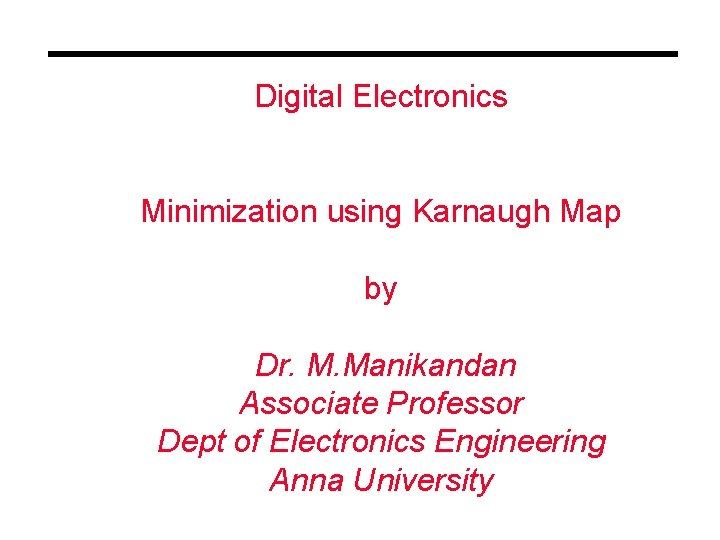Digital Electronics Minimization using Karnaugh Map by Dr. M. Manikandan Associate Professor Dept of Electronics Engineering Anna UniversityOverview ° K-maps: an alternate approach to representing Boolean functions ° K-map representation can be used to minimize Boolean functions ° Easy conversion from truth table to K-map to minimized SOP representation. ° Simple rules (steps) used to perform minimization ° Leads to minimized SOP representation. • Much faster and more efficient than previous minimization techniques with Boolean algebra.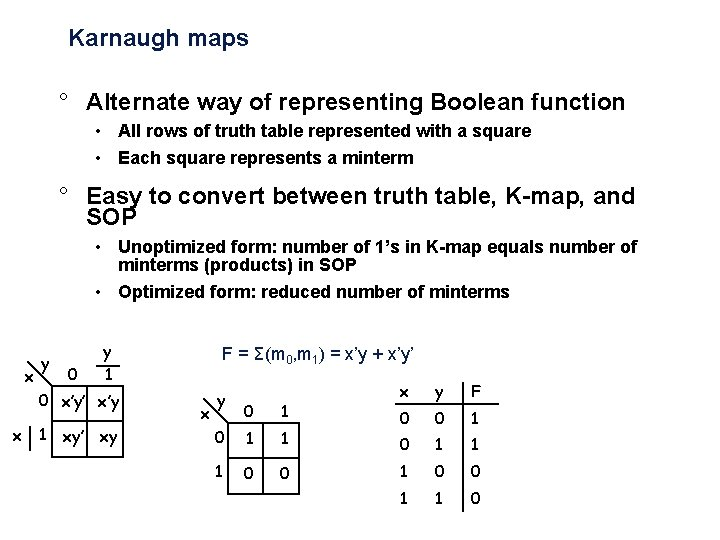Karnaugh maps ° Alternate way of representing Boolean function • All rows of truth table represented with a square • Each square represents a minterm ° Easy to convert between truth table, K-map, and SOP • Unoptimized form: number of 1’s in K-map equals number of minterms (products) in SOP • Optimized form: reduced number of minterms x y 0 y 1 0 x’y’ x’y x 1 xy’ xy F = Σ(m 0, m 1) = x’y + x’y’ x y 0 1 1 1 0 0 x y F 0 0 1 1 1 0 0 1 1 0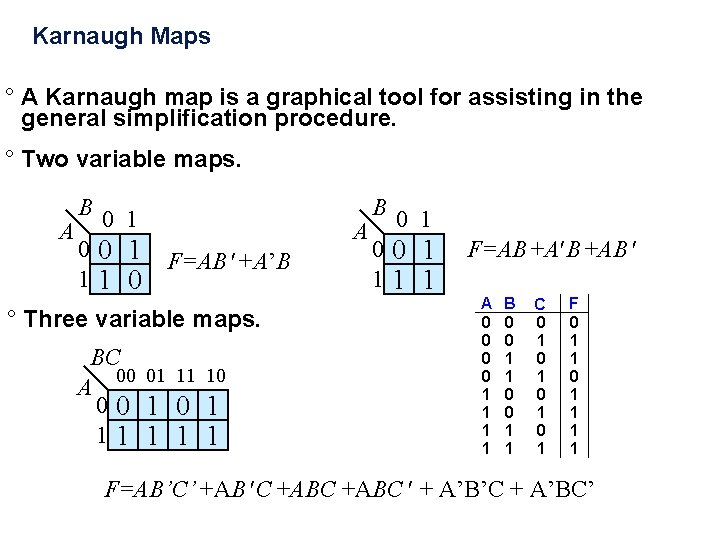Karnaugh Maps ° A Karnaugh map is a graphical tool for assisting in the general simplification procedure. ° Two variable maps. B 0 1 A 00 1 11 0 F=AB +A’B B 0 1 A 00 1 11 1 F=AB +AB ° Three variable maps. BC 00 01 11 10 A 00 1 11 1 + A 0 0 1 1 B 0 0 1 1 C 0 1 0 1 F 0 1 1 1 1 F=AB’C’ +AB C +ABC + A’B’C + A’BC’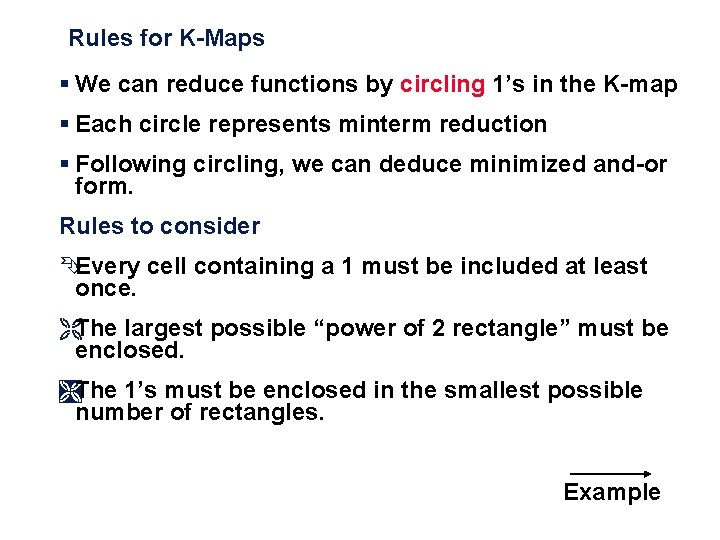Rules for K-Maps § We can reduce functions by circling 1’s in the K-map § Each circle represents minterm reduction § Following circling, we can deduce minimized and-or form. Rules to consider ÊEvery cell containing a 1 must be included at least once. ËThe largest possible “power of 2 rectangle” must be enclosed. ÌThe 1’s must be enclosed in the smallest possible number of rectangles. Example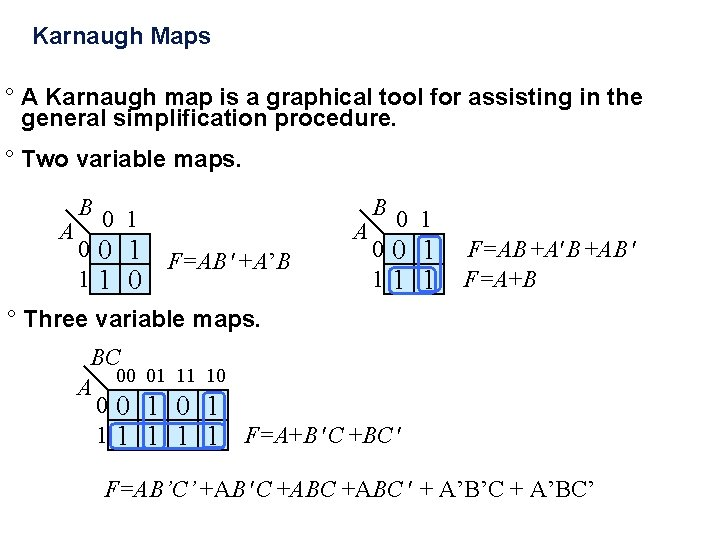Karnaugh Maps ° A Karnaugh map is a graphical tool for assisting in the general simplification procedure. ° Two variable maps. B 0 1 A 00 1 11 0 F=AB +A’B B 0 1 A 00 1 11 1 F=AB +AB F=A+B ° Three variable maps. BC 00 01 11 10 A 00 1 1 1 1 F=A+B C +BC F=AB’C’ +AB C +ABC + A’B’C + A’BC’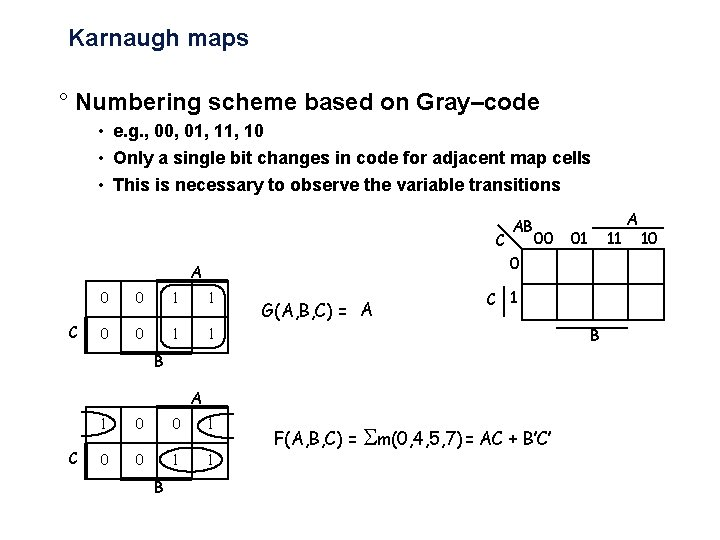Karnaugh maps ° Numbering scheme based on Gray–code • e. g. , 00, 01, 10 • Only a single bit changes in code for adjacent map cells • This is necessary to observe the variable transitions C 00 0 0 1 1 G(A, B, C) = A C 1 B B A C 1 0 0 1 1 B 11 01 0 A C AB F(A, B, C) = m(0, 4, 5, 7) = AC + B’C’ A 10More Karnaugh Map Examples ° Examples a b 0 1 0 0 1 1 0 1 f=a ab c 00 01 11 10 0 1 0 1 1 1 cout = ab + bc + ac a b 0 1 1 1 0 0 g = b' ab c 00 01 11 10 0 1 1 1 0 0 1 1 f=a 1. Circle the largest groups possible. 2. Group dimensions must be a power of 2. 3. Remember what circling means!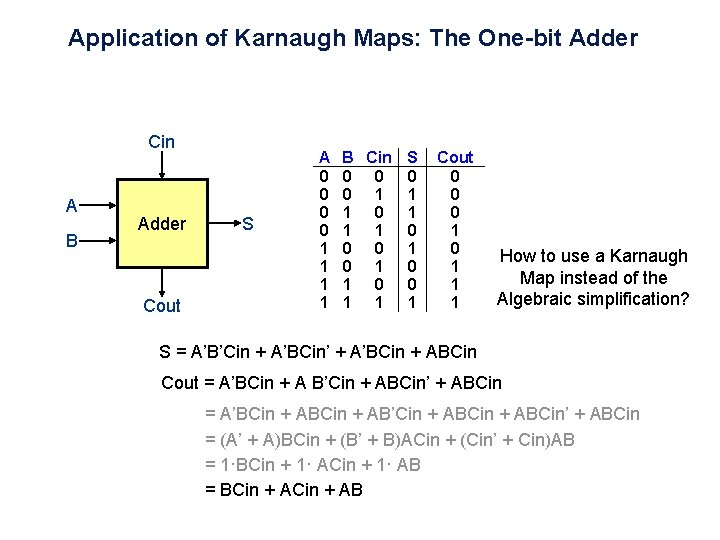Application of Karnaugh Maps: The One-bit Adder Cin A B Adder Cout S + A 0 0 1 1 B Cin S 0 0 1 1 1 0 0 0 1 0 1 0 0 1 1 1 Cout 0 0 0 1 1 1 How to use a Karnaugh Map instead of the Algebraic simplification? S = A’B’Cin + A’BCin’ + A’BCin + ABCin Cout = A’BCin + A B’Cin + ABCin’ + ABCin = A’BCin + AB’Cin + ABCin’ + ABCin = (A’ + A)BCin + (B’ + B)ACin + (Cin’ + Cin)AB = 1·BCin + 1· AB = BCin + ABApplication of Karnaugh Maps: The One-bit Adder Cin A Adder B S Cout B A 0 0 1 1 1 Cin Karnaugh Map for Cout Now we have to cover all the 1 s in the Karnaugh Map using the largest rectangles and as few rectangles as we can.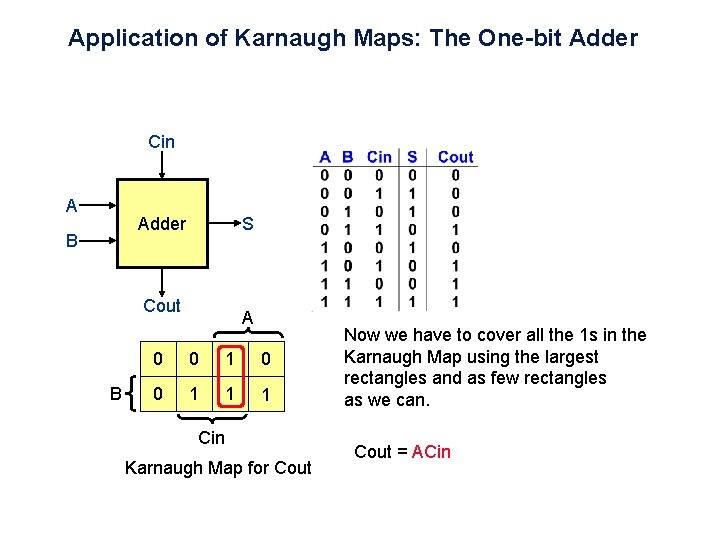Application of Karnaugh Maps: The One-bit Adder Cin A Adder B S Cout B A 0 0 1 1 1 Cin Karnaugh Map for Cout Now we have to cover all the 1 s in the Karnaugh Map using the largest rectangles and as few rectangles as we can. Cout = ACin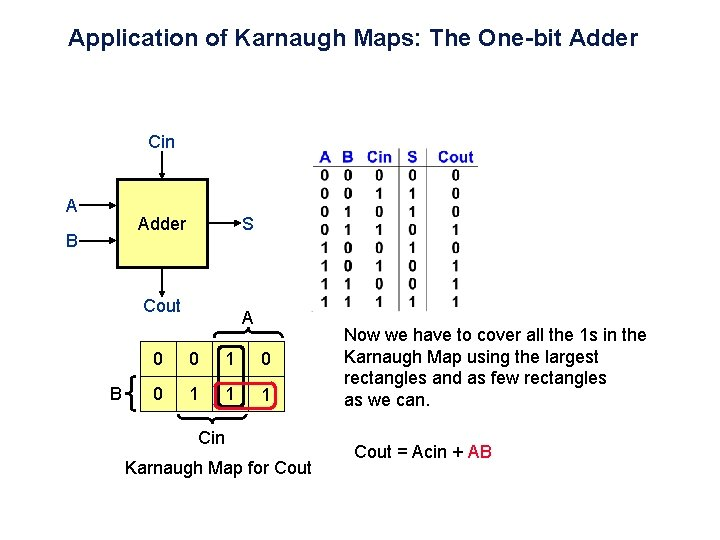Application of Karnaugh Maps: The One-bit Adder Cin A Adder B S Cout B A 0 0 1 1 1 Cin Karnaugh Map for Cout Now we have to cover all the 1 s in the Karnaugh Map using the largest rectangles and as few rectangles as we can. Cout = Acin + AB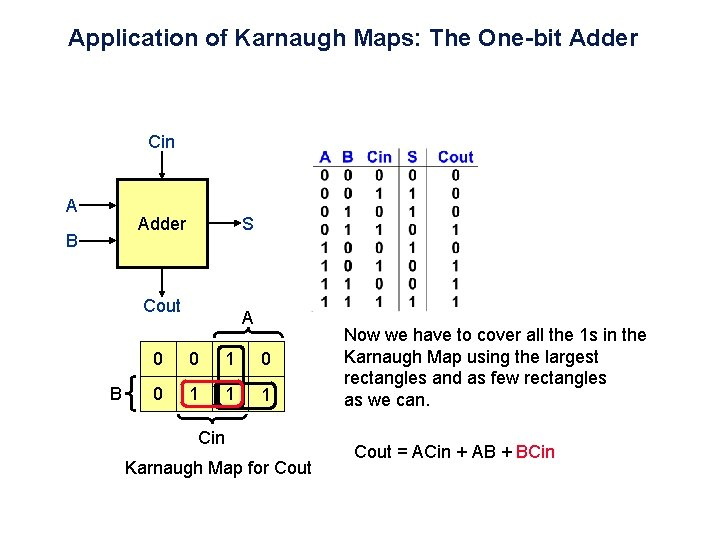Application of Karnaugh Maps: The One-bit Adder Cin A Adder B S Cout B A 0 0 1 1 1 Cin Karnaugh Map for Cout Now we have to cover all the 1 s in the Karnaugh Map using the largest rectangles and as few rectangles as we can. Cout = ACin + AB + BCin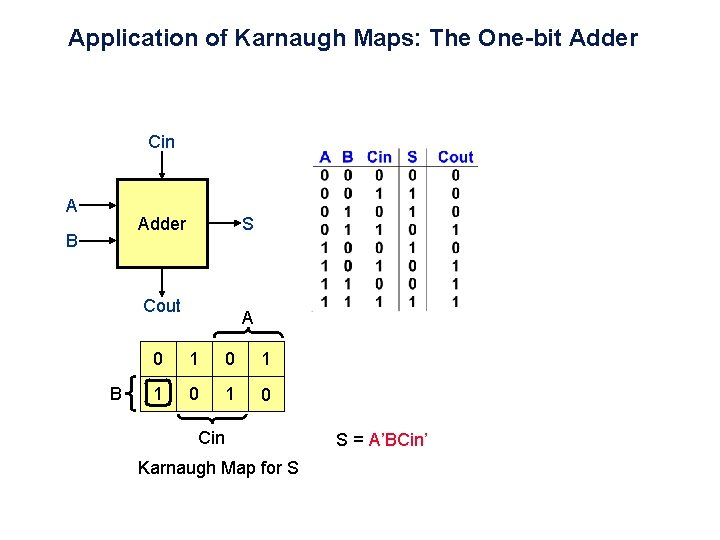Application of Karnaugh Maps: The One-bit Adder Cin A Adder B S Cout B A 0 1 1 0 Cin Karnaugh Map for S S = A’BCin’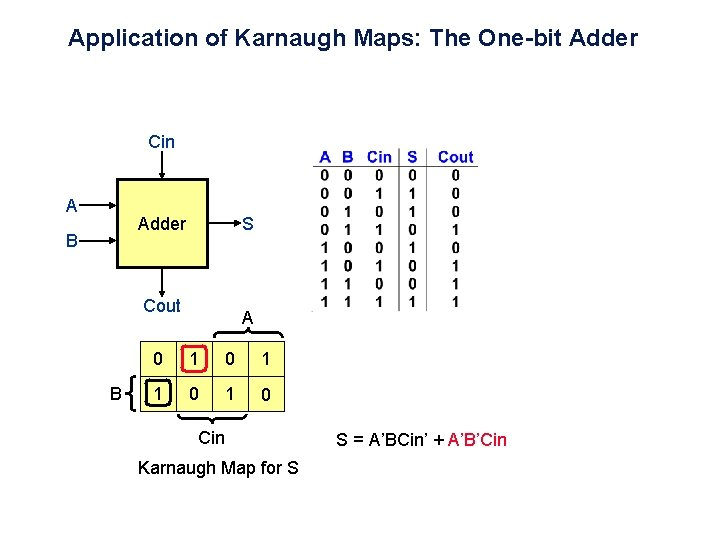Application of Karnaugh Maps: The One-bit Adder Cin A Adder B S Cout B A 0 1 1 0 Cin Karnaugh Map for S S = A’BCin’ + A’B’Cin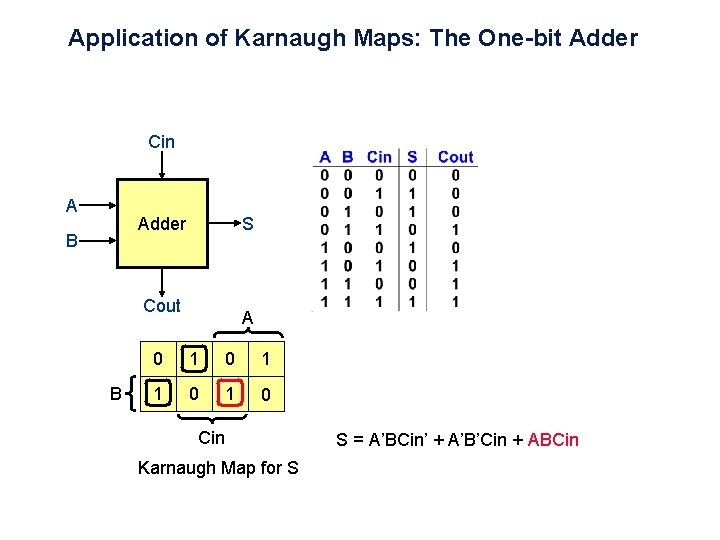Application of Karnaugh Maps: The One-bit Adder Cin A Adder B S Cout B A 0 1 1 0 Cin Karnaugh Map for S S = A’BCin’ + A’B’Cin + ABCin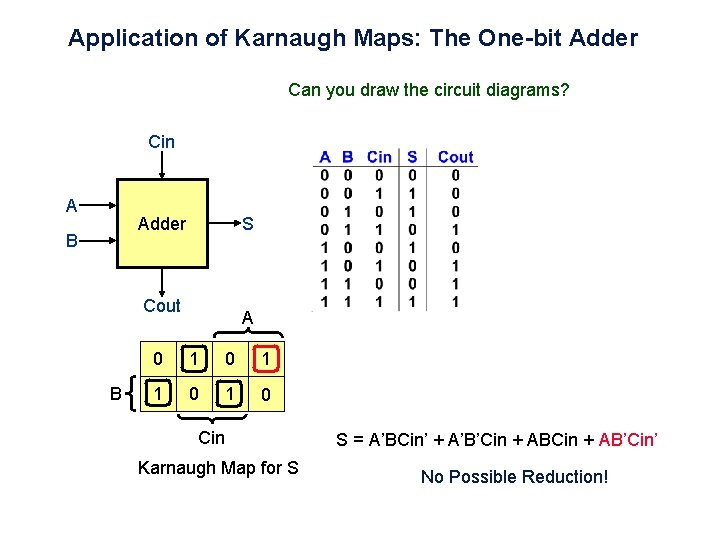Application of Karnaugh Maps: The One-bit Adder Can you draw the circuit diagrams? Cin A Adder B S Cout B A 0 1 1 0 Cin Karnaugh Map for S S = A’BCin’ + A’B’Cin + AB’Cin’ No Possible Reduction!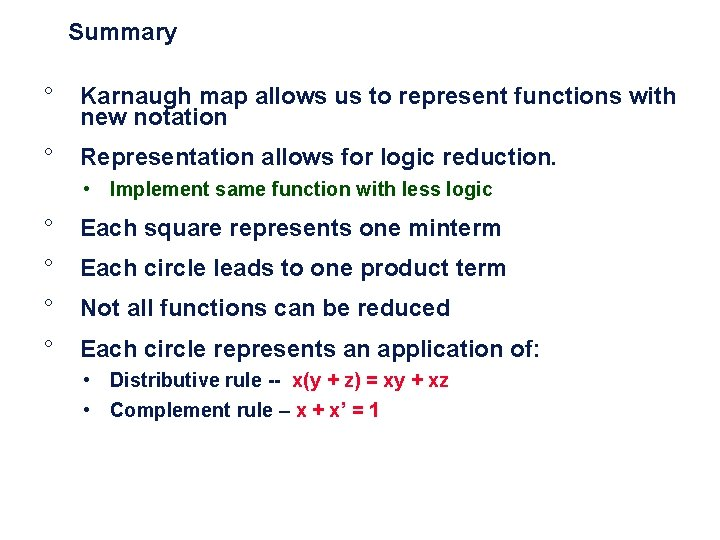Summary ° Karnaugh map allows us to represent functions with new notation ° Representation allows for logic reduction. • Implement same function with less logic ° Each square represents one minterm ° Each circle leads to one product term ° Not all functions can be reduced ° Each circle represents an application of: • Distributive rule -- x(y + z) = xy + xz • Complement rule – x + x’ = 1Karnaugh Maps and Don’t CaresOverview ° Karnaugh maps with four inputs • Same basic rules as three input K-maps ° Understanding prime implicants • Related to minterms ° Covering all implicants ° Using Don’t Cares to simplify functions • Don’t care outputs are undefined ° Summarizing Karnaugh maps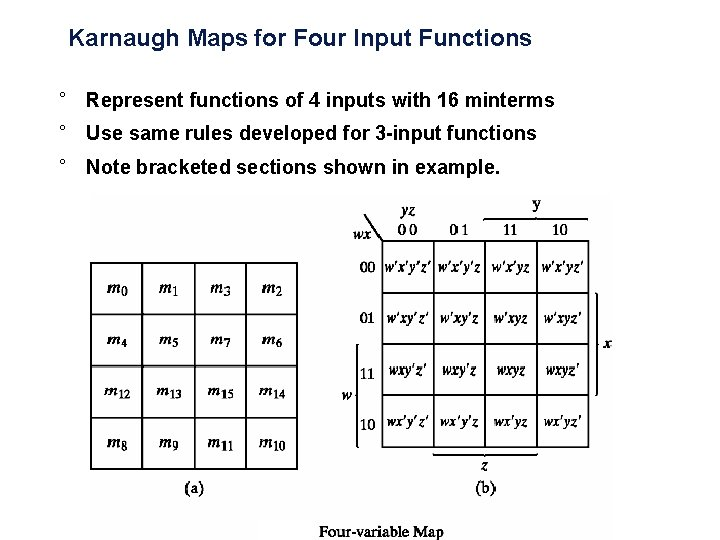Karnaugh Maps for Four Input Functions ° Represent functions of 4 inputs with 16 minterms ° Use same rules developed for 3 -input functions ° Note bracketed sections shown in example.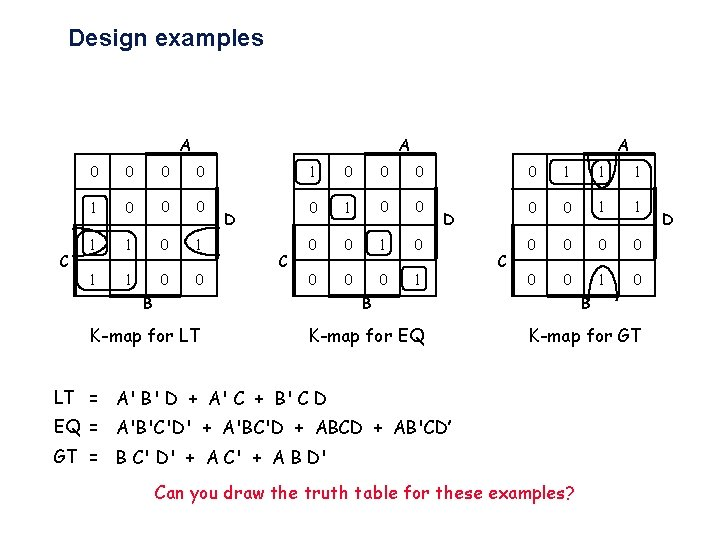Design examples A A C 0 0 1 0 0 0 1 1 1 0 0 D C A 1 0 0 0 0 1 D C 0 1 1 1 0 0 0 0 1 0 B B B K-map for LT K-map for EQ K-map for GT LT = A' B' D + A' C + B' C D EQ = A'B'C'D' + A'BC'D + ABCD + AB'CD’ GT = B C' D' + A C' + A B D' Can you draw the truth table for these examples? DPhysical Implementation A B C ° Step 1: Truth table D ° Step 2: K-map ° Step 3: Minimized sum-ofproducts EQ ° Step 4: Physical implementation with gates A C 1 0 0 0 0 1 B K-map for EQ DKarnaugh Maps ° Four variable maps. CD 00 01 11 10 AB 00 01 11 10 0 1 1 1 0 0 0 1 1 1 F=A BC +A CD +ABC +AB C F=BC +CD + AC+ AD ° Need to make sure all 1’s are covered ° Try to minimize total product terms. ° Design could be implemented using NANDs and NORs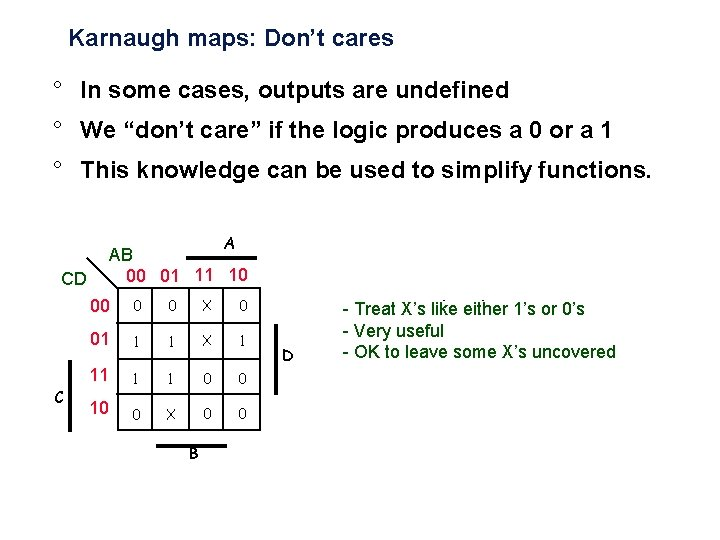Karnaugh maps: Don’t cares ° In some cases, outputs are undefined ° We “don’t care” if the logic produces a 0 or a 1 ° This knowledge can be used to simplify functions. A CD C AB 00 01 11 10 00 0 0 X 0 01 1 1 X 1 11 1 1 0 0 10 0 X 0 0 B D - Treat X’s like either 1’s or 0’s - Very useful - OK to leave some X’s uncovered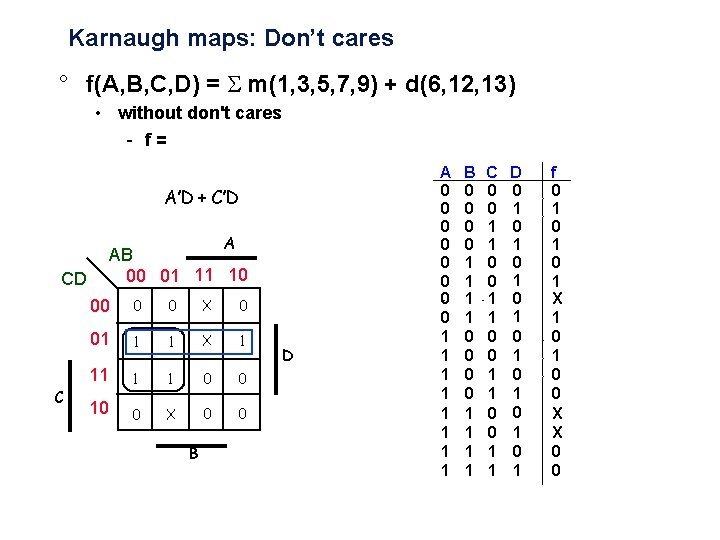Karnaugh maps: Don’t cares ° f(A, B, C, D) = m(1, 3, 5, 7, 9) + d(6, 12, 13) • without don't cares - f = A’D + C’D A CD AB 00 01 11 10 00 0 X 0 0 + C 01 1 1 X 1 11 1 1 0 0 10 0 X 0 0 B D A 0 0 0 0 1 1 1 1 + B 0 0 0 0 1 1 1 1 C 0 0 1 1 D 0 1 0 1 f 0 1 0 1 X 1 0 0 X X 0 0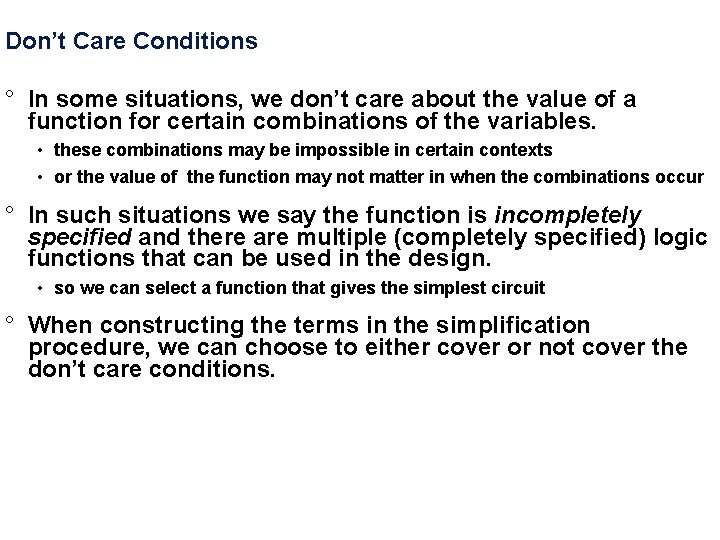Don’t Care Conditions ° In some situations, we don’t care about the value of a function for certain combinations of the variables. • these combinations may be impossible in certain contexts • or the value of the function may not matter in when the combinations occur ° In such situations we say the function is incompletely specified and there are multiple (completely specified) logic functions that can be used in the design. • so we can select a function that gives the simplest circuit ° When constructing the terms in the simplification procedure, we can choose to either cover or not cover the don’t care conditions.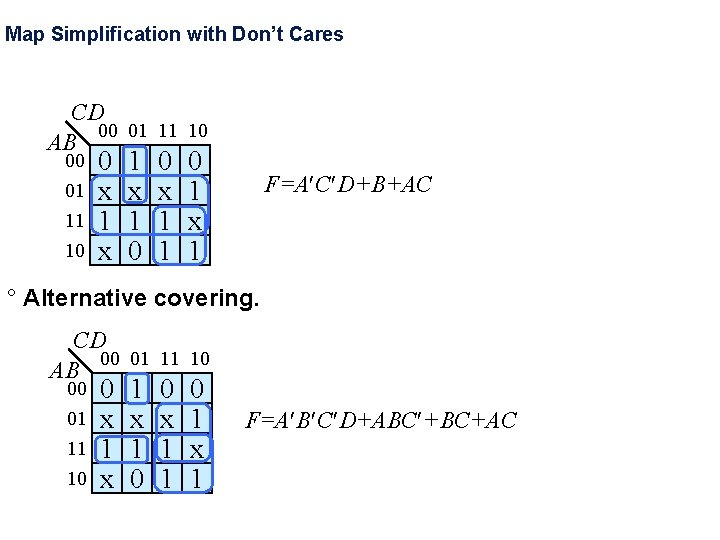Map Simplification with Don’t Cares CD 00 01 11 10 AB 00 01 11 10 0 x 1 x 1 0 0 x 1 1 0 1 x 1 F=A C D+B+AC ° Alternative covering. CD 00 01 11 10 AB 00 01 11 10 0 x 1 x 1 0 0 x 1 1 0 1 x 1 F=A B C D+ABC +BC+ACKarnaugh maps: don’t cares (cont’d) ° f(A, B, C, D) = m(1, 3, 5, 7, 9) + d(6, 12, 13) • f = A'D + B'C'D • f = without don't cares with don't cares A'D + C'D A C 0 0 X 0 1 1 X 1 1 1 0 0 0 X 0 0 B D by using don't care as a "1" a 2 -cube can be formed rather than a 1 -cube to cover this node don't cares can be treated as 1 s or 0 s depending on which is more advantageous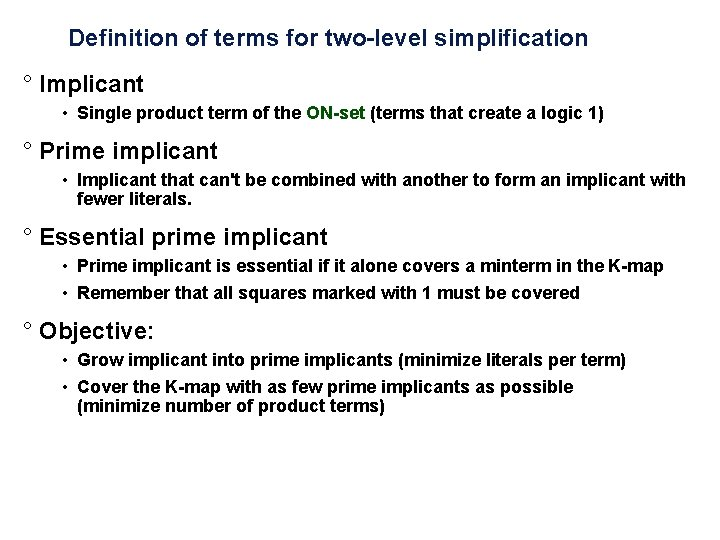Definition of terms for two-level simplification ° Implicant • Single product term of the ON-set (terms that create a logic 1) ° Prime implicant • Implicant that can't be combined with another to form an implicant with fewer literals. ° Essential prime implicant • Prime implicant is essential if it alone covers a minterm in the K-map • Remember that all squares marked with 1 must be covered ° Objective: • Grow implicant into prime implicants (minimize literals per term) • Cover the K-map with as few prime implicants as possible (minimize number of product terms)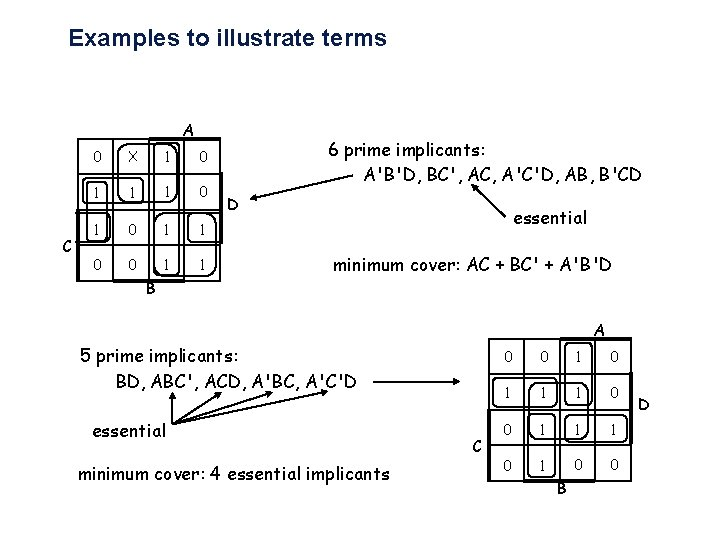Examples to illustrate terms A C 0 X 1 0 1 1 0 0 1 1 B 6 prime implicants: A'B'D, BC', AC, A'C'D, AB, B'CD D essential minimum cover: AC + BC' + A'B'D A 5 prime implicants: BD, ABC', ACD, A'BC, A'C'D essential minimum cover: 4 essential implicants C 0 0 1 1 1 0 0 B D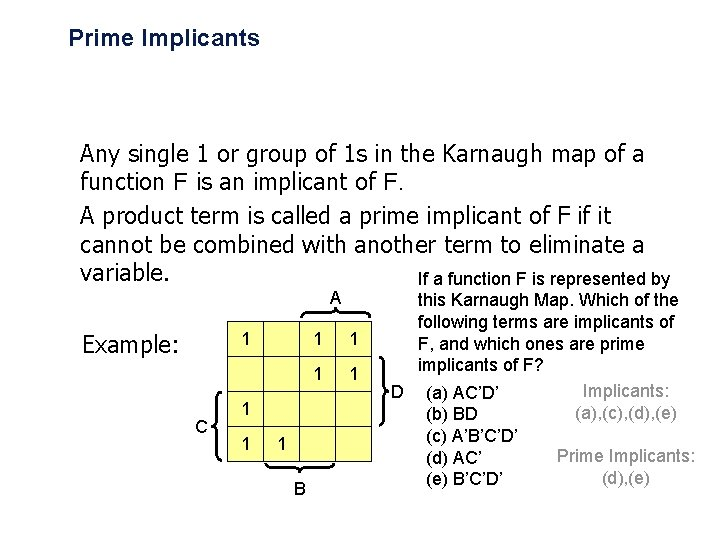Prime Implicants Any single 1 or group of 1 s in the Karnaugh map of a function F is an implicant of F. A product term is called a prime implicant of F if it cannot be combined with another term to eliminate a variable. If a function F is represented by A Example: 1 C 1 1 1 B 1 1 this Karnaugh Map. Which of the following terms are implicants of F, and which ones are prime implicants of F? Implicants: D (a) AC’D’ (a), (c), (d), (e) (b) BD (c) A’B’C’D’ Prime Implicants: (d) AC’ (d), (e) B’C’D’Essential Prime Implicants A product term is an essential prime implicant if there is a minterm that is only covered by that prime implicant. - The minimal sum-of-products form of F must include all the essential prime implicants of F.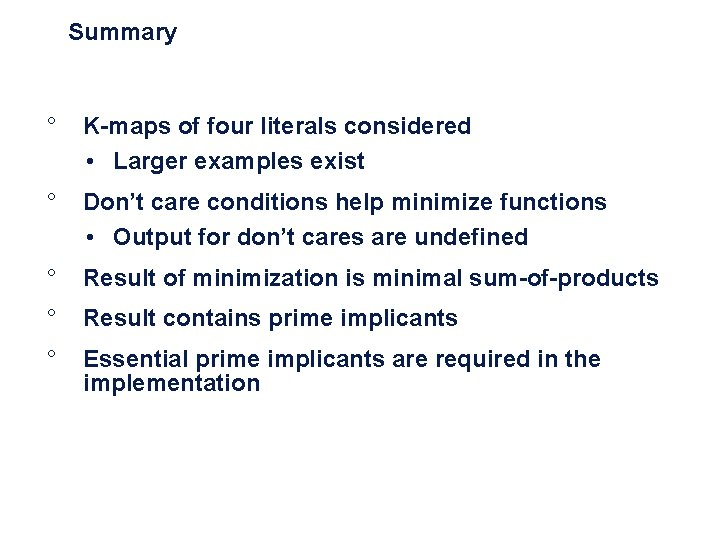Summary ° K-maps of four literals considered • Larger examples exist ° Don’t care conditions help minimize functions • Output for don’t cares are undefined ° Result of minimization is minimal sum-of-products ° Result contains prime implicants ° Essential prime implicants are required in the implementation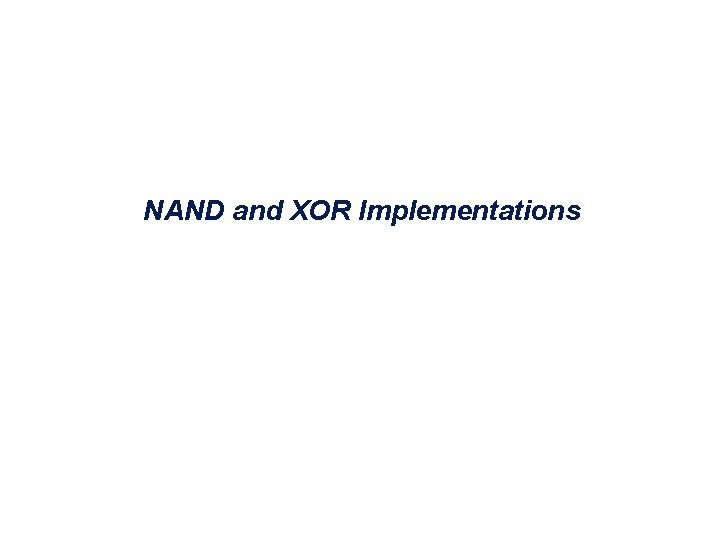NAND and XOR Implementations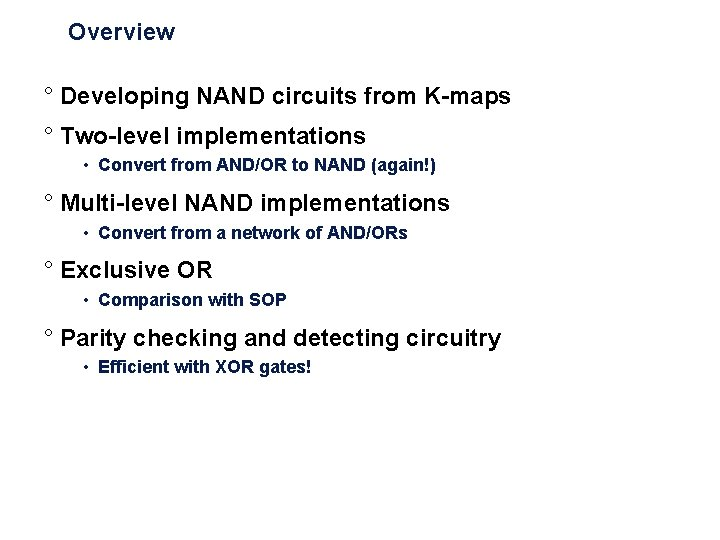Overview ° Developing NAND circuits from K-maps ° Two-level implementations • Convert from AND/OR to NAND (again!) ° Multi-level NAND implementations • Convert from a network of AND/ORs ° Exclusive OR • Comparison with SOP ° Parity checking and detecting circuitry • Efficient with XOR gates!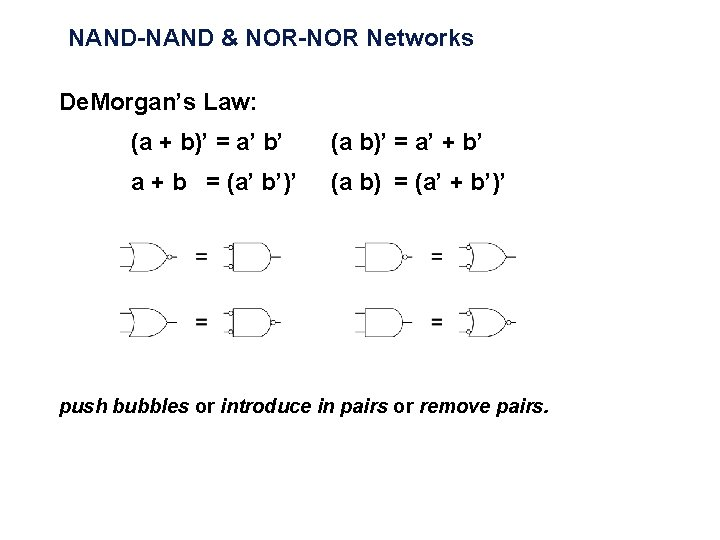NAND-NAND & NOR-NOR Networks De. Morgan’s Law: (a + b)’ = a’ b’ (a b)’ = a’ + b’ a + b = (a’ b’)’ (a b) = (a’ + b’)’ push bubbles or introduce in pairs or remove pairs.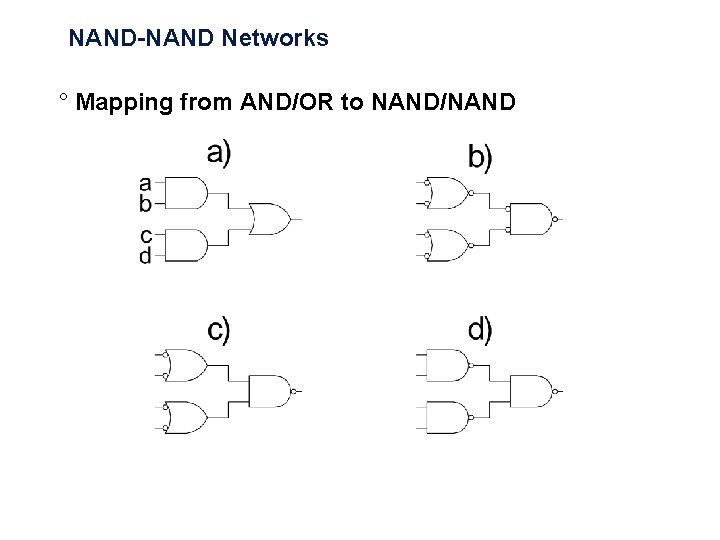NAND-NAND Networks ° Mapping from AND/OR to NAND/NAND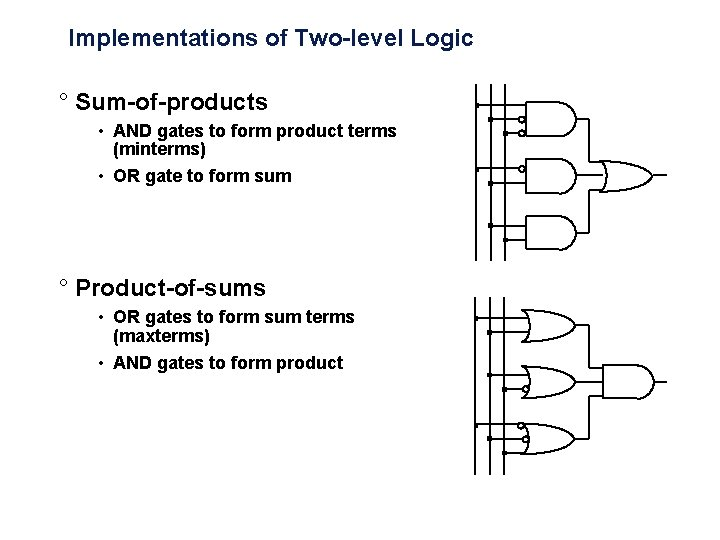Implementations of Two-level Logic ° Sum-of-products • AND gates to form product terms (minterms) • OR gate to form sum ° Product-of-sums • OR gates to form sum terms (maxterms) • AND gates to form product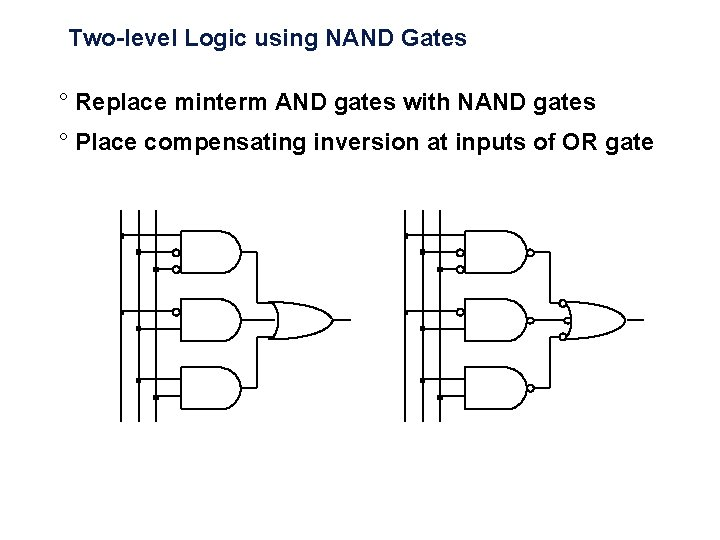Two-level Logic using NAND Gates ° Replace minterm AND gates with NAND gates ° Place compensating inversion at inputs of OR gate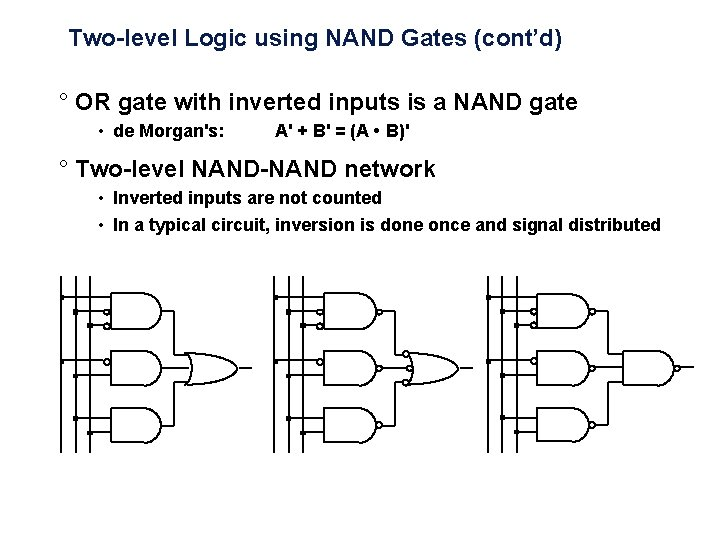Two-level Logic using NAND Gates (cont’d) ° OR gate with inverted inputs is a NAND gate • de Morgan's: A' + B' = (A • B)' ° Two-level NAND-NAND network • Inverted inputs are not counted • In a typical circuit, inversion is done once and signal distributedConversion Between Forms ° Convert from networks of ANDs and ORs to networks of NANDs and NORs • Introduce appropriate inversions ("bubbles") ° Each introduced "bubble" must be matched by a corresponding "bubble" • Conservation of inversions • Do not alter logic function ° Example: AND/OR to NAND/NAND A A B B C D Z C D NAND ZConversion Between Forms (cont’d) ° Example: verify equivalence of two forms A A B B C D Z C D NAND Z = [ (A • B)' • (C • D)' ]' = [ (A' + B') • (C' + D') ]' = [ (A' + B')' + (C' + D')' ] = (A • B) + (C • D) ü ZConversion to NAND Gates ° Start with SOP (Sum of Products) • circle 1 s in K-maps ° Find network of OR and AND gates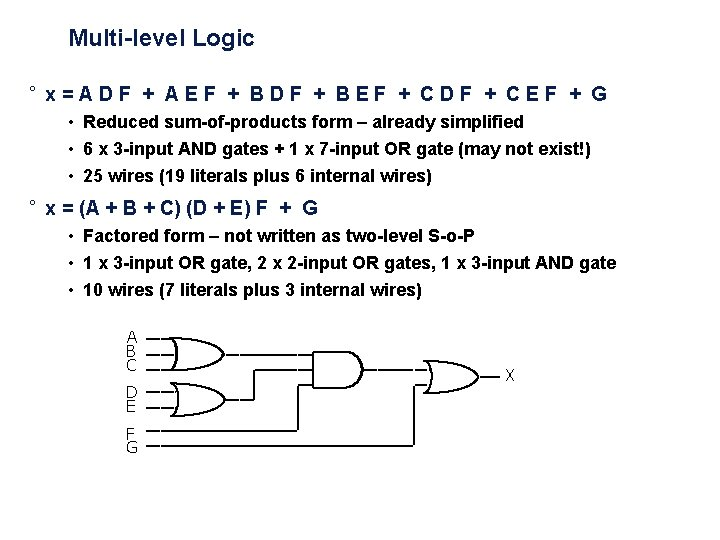Multi-level Logic ° x = A D F + A E F + B D F + B E F + C D F + C E F + G • Reduced sum-of-products form – already simplified • 6 x 3 -input AND gates + 1 x 7 -input OR gate (may not exist!) • 25 wires (19 literals plus 6 internal wires) ° x = (A + B + C) (D + E) F + G • Factored form – not written as two-level S-o-P • 1 x 3 -input OR gate, 2 x 2 -input OR gates, 1 x 3 -input AND gate • 10 wires (7 literals plus 3 internal wires) A B C D E F G X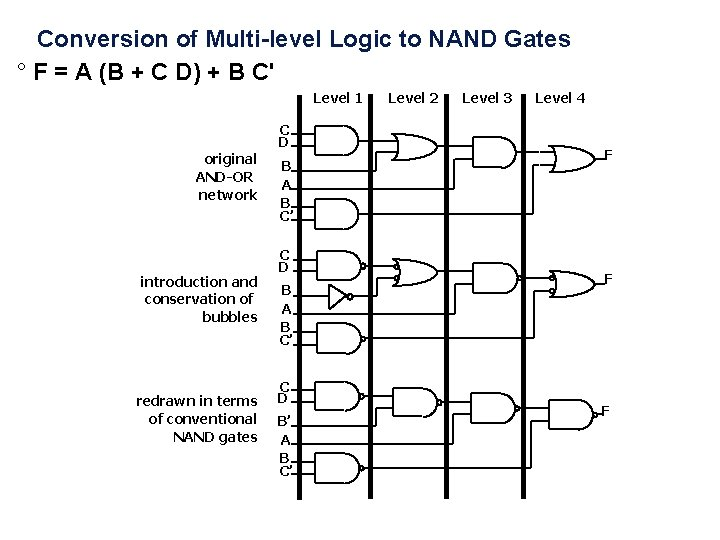Conversion of Multi-level Logic to NAND Gates ° F = A (B + C D) + B C' Level 1 C D original AND-OR network introduction and conservation of bubbles redrawn in terms of conventional NAND gates B A B C’ C D B’ A B C’ Level 2 Level 3 Level 4 F F FConversion Between Forms ° Example A A (a) B C D X B C D F Add double bubbles at inputs Original circuit A A (c) B C D’ X (b) F X’ Distribute bubbles some mismatches B C D’ X’ Insert inverters to fix mismatches F (d)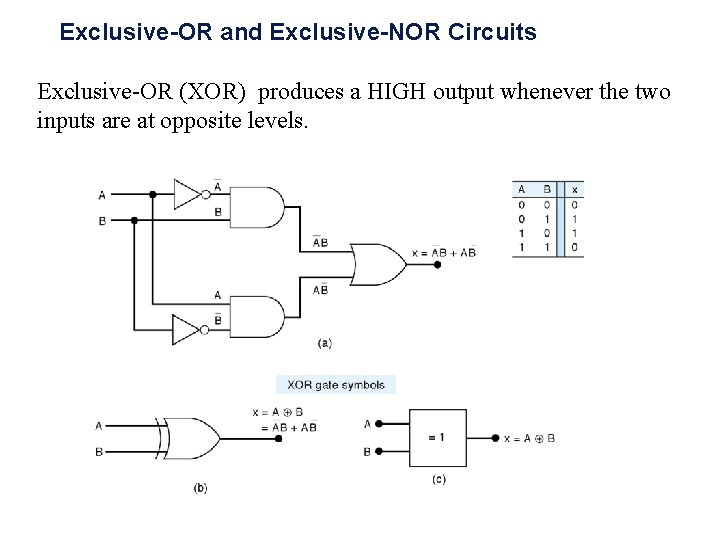Exclusive-OR and Exclusive-NOR Circuits Exclusive-OR (XOR) produces a HIGH output whenever the two inputs are at opposite levels.Exclusive-NOR Circuits Exclusive-NOR (XNOR) : Exclusive-NOR (XNOR) produces a HIGH output whenever the two inputs are at the same level.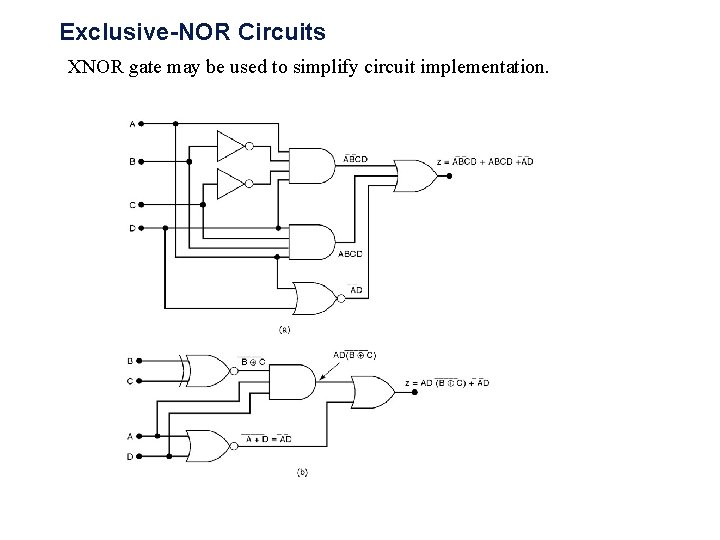Exclusive-NOR Circuits XNOR gate may be used to simplify circuit implementation.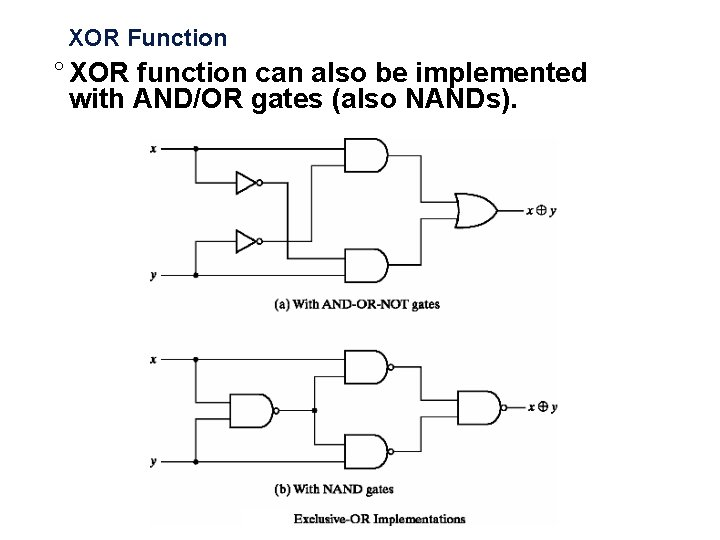XOR Function ° XOR function can also be implemented with AND/OR gates (also NANDs).XOR Function ° Even function – even number of inputs are 1. ° Odd function – odd number of inputs are 1.Parity Generation and Checking XOR gates used to implement the parity generator and the parity checker for an even-parity system.Summary ° Follow rules to convert between AND/OR representation and symbols ° Conversions are based on De. Morgan’s Law ° NOR gate implementations are also possible ° XORs provide straightforward implementation for some functions ° Used for parity generation and checking • XOR circuits could also be implemented using AND/Ors